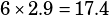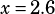Can you do Test 6 #36 from the calculator section

This question rocks!

Since you have data for all the rocks Andrew collected, calculate the average mass of Andrew’s rocks.If the average mass of Maria’s rocks is 0.1 kg greater, then, Maria’s rocks must have an average mass of 2.9 kg.

Now, as in most average questions, you’re going to need to deal with sums. If Maria has 6 rocks, with an average mass of 2.9 kg, then the total mass of her rocks iskg. Use that to solve for x: